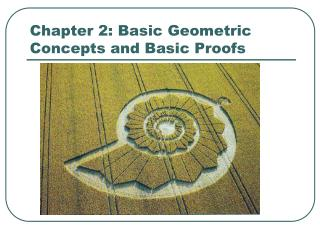# Chapter 2: Basic Geometric Concepts and Basic Proofs - PowerPoint PPT PresentationDownload PresentationChapter 2: Basic Geometric Concepts and Basic Proofs

Chapter 2: Basic Geometric Concepts and Basic ProofsDownload Presentation## Chapter 2: Basic Geometric Concepts and Basic Proofs

- - - - - - - - - - - - - - - - - - - - - - - - - - - E N D - - - - - - - - - - - - - - - - - - - - - - - - - - -
##### Presentation Transcript

1. Chapter 2: Basic Geometric Concepts and Basic Proofs

2. 2.1 Perpendicularity Symbol for perpendicular: Definition: Lines, rays, or segments that intersect at right angles are perpendicular.

3. **In the little mark inside the angle (L) indicates <DEF is a right angle. ** E J a F D G b H M Perpendicular: • Lines, Rays, or Segments that intersect at right angles • Examples: **This also means m<DEF = 90°**

4. A In it appears that BUT, we must be given this or be able to prove it, you CANNOT ASSUME it. C B Can we assume a right angle?? • NO!!!!! • Therefore, you cannot assume perpendicularity from a diagram either.

5. J O 4x x M K • Given: is 4 times as large as Find: **Since , ** Example 1:

6. D A Given: Prove: C B Reason Statement Example 2: 1. Given 2. If 2 segs. are perpendicular, then they form a right angle 2. <ABC is right angle <DCB is right angle 3. If 2 angles are right angles, then they are congruent. (or just Theorem 1)

7. Examples of Perpendicularity The x-y axes Quadrant Numbers?

8. Examples of Perpendicularity “T-Square”

9. Perpendicular Planes

10. Triangle Altitudes

11. Definition: Two intersecting non-parallel lines are called oblique lines.

12. Definition: Two non-intersecting non-parallel lines are called skew lines.